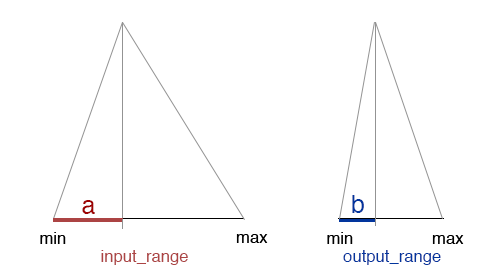### OSLFloat Remapping

#### Introduction

It is often necessary to remap, or convert, floating point values from one range to another range. For example, if the `resultF` output of a PxrFractal node is used to control the `diffuseGain` of a material node the values may require conversion from their original range of 0.0 - 1.0 to a smaller range of, say, 0.2 - 0.8.

This tutorial explains one way of performing a remapping. The diagram shown below represents two ranges of numbers as the bases of two triangles.If the `input_range` has a `min` value of 0.0 and a `max` value of 1.0 it's range would be 1.0. If the `output_range` has a `min` value of 0.2 and a `max` value of 0.8 it's range would be 0.6. For a specific input value, represented by the red line, to be remapped to an output value, represented by the blue line, the following must be true.     ```a/input_range = b/output_range``` Consequently, the value of b would be,     ```b = a/input_range * output_range``` A remapped value would be,     ```remapped = a/input_range * output_range + output_min``` For example, if the input value is 0.35 it's remapped output value would be,     `remapped = 0.35/1.0 * 0.6 + 0.2`     `remapped = 0.41` Listing 1 uses the arithmetic shown above to implement a float remap shader.

Listing 1

 ```shader FRemap( float input = 0, float input_min = 0, float input_max = 1, float output_min = 0, float output_max = 1, output float resultF = 0) { float input_range = fabs(input_max - input_min); float output_range = fabs(output_max - output_min); float a = fabs(output_min - input); resultF = a / input_range * output_range + output_min; }```